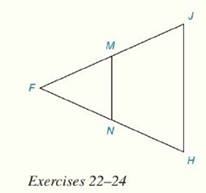Chapter 4.CR, Problem 22CRElementary Geometry For College St...

7th Edition
Alexander + 2 others
ISBN: 9781337614085

Solutions

Chapter
SectionElementary Geometry For College St...

7th Edition
Alexander + 2 others
ISBN: 9781337614085
Textbook Problem

Review ExercisesIn Review Exercises 22 to 24, M and N are the midpoints of F J - and F H - respectively.Given: Isosceles ∆ F J H with F J - ≅ F H - F M = 2 y + 3 N H = 5 y - 9 J H = 2 y Find: The perimeter of ∆ F M N

To determine

To Find:

The perimeter of an isosceles triangle using the information provided.

Explanation

Theorem on triangle:

The line segment that joins the midpoints of two sides of a triangle is parallel to the third side and has a length equal to one-half the length of the third side.

That is, ABC with midpoints M and N of AB- and AC-, respectively. Then MN-BC-. It can be concluded that BC=12(MN)

Calculation:

It is given that FJH is an isosceles triangle with M and N are the midpoints of FJ- and FH- respectively.

Also given that FJ-FH-, FM=2y+3, NH=5y-9 and JH=2y.

The perimeter of FMN is FM+MN+FN.

Since FJ-FH- and M and N are the midpoints of FJ- and FH-, FN=NH=FM=MJ

Still sussing out bartleby?

Check out a sample textbook solution.

See a sample solution

The Solution to Your Study Problems

Bartleby provides explanations to thousands of textbook problems written by our experts, many with advanced degrees!

Get Started

Define the terms population and sample, and explain the role of each in a research study.

Essentials of Statistics for The Behavioral Sciences (MindTap Course List)

Prove that, for all x1, 2x31x

Calculus (MindTap Course List)

In Problems 21-26, graph each function. 24.

Mathematical Applications for the Management, Life, and Social Sciences

In Exercises 5-8, graph the given function or equation. y={12xif1x1x1if1x3

Finite Mathematics and Applied Calculus (MindTap Course List)

True or False: Let . Then f(x, y) is a joint probability function.

Study Guide for Stewart's Multivariable Calculus, 8th